Behavior Driven Development, or short, BDD is a software development methodology that relies on examples to define the behavior of a system.

In this article, we’re going to learn the bases of BDD, see what tools are available, and see BDD in action through simple examples in Visual Studio and C#.

Let’s start.

## What Problems Does BDD Solve?

There is often a communication gap between those writing the software and the others involved in the development process. In other words, programmers and non-programmers do not speak the same language when talking about the product (probably in general).

Behavior-Driven Development bridges the gap using Gherkin, natural language syntax, and available tools.

## Gherkin – The Language of BDD

The language of BDD is Gherkin, a natural-language syntax through which we describe the required behavior of a system.

For example, if we want to describe a test case for a function that sums two numbers in a natural language, it might be something like this:

“If we add a number 5 to a number 3, the result should be 8.”

Gherkin uses a “Given-When-Then” pattern, and if we follow it, the correct formulation is:

“Given we have a number 3

When we add the number 5

Then the result is 8.”

This sentence is almost as easy to read as the “natural” way of saying the same thing.  But saying it this way gives our BDD tool a format that enables it to parse the information required to test our functionality. The key ingredient for this is the usage of keywords – Given, And, When, and Then.

## BDD in .NET – SpecFlow

There are other tools available for BDD, but in this article, we will focus on SpecFlow.

SpecFlow is a free, open-source tool that utilizes C# and .NET methods. It integrates into Visual Studio and provides an easy way to feature files through its Gherking editor. It also provides a simple way to generate test code from the feature description.

The SpecFlow team brought us additional tools that extend SpecFlow functionalities.

SpecFlow+LivingDoc is a set of collaboration tools that allows us to generate and share documentation with the team.

SpecMap is a story mapping tool made for Team Services. It maps stories directly within Azure DevOps and links them to the project backlog.

SpecFlow+Runner is a dedicated test runner for SpecFlow that integrates directly into Visual Studio and provides test execution from the command line. It does not support .NET 6 since the development has been discontinued. The last available version SpecFlow+Runner is 3.9.31.

## SpecFlow for Visual Studio

To use SpecFlow in our solutions, we need to install SpecFlow for the Visual Studio extension. We can do it directly in the Visual Studio extensions manager.Now we can add a SpecFlow project to our solution.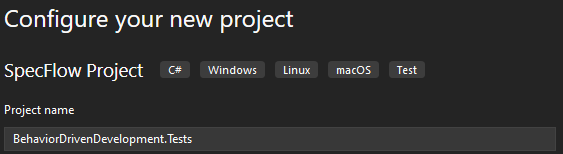## SpecFlow in Action

Although it might not be in the spirit of BDD to write the functionality first, we will make this exception so we can focus on BDD features.

So, let’s define our `Helpers` class:

```public static class Helpers
{
public static int SumTwoNumbers(int a, int b)
{
return a + b;
}

public static int SubtractTwoNumbers(int a, int b)
{
return a - b;
}

public static int GetAverage(int a, int b, int c)
{
return (int)new int[] { a, b, c }.Average();
}
}```

Here we defined methods to get sum, subtract, and average results for the input numbers.

Let’s also define the `Extensions` class:

```public static class Extensions
{
public static int GetWordCount(this string input)
{
return input.Split(' ', StringSplitOptions.RemoveEmptyEntries).Length;
}

public static int GetCharCount(this string input)
{
return input.Replace(" ", "").Length;
}
}```

Here we defined a method `GetWordCount()`, which returns the number of words in a string. And a method `GetCharCount()`, which returns a number of characters in a string without the spaces.

### SpecFlow Files and Helpers

Now we are ready to add the SpecFlow feature file by selecting it from the SpecFlow templates in Visual Studio.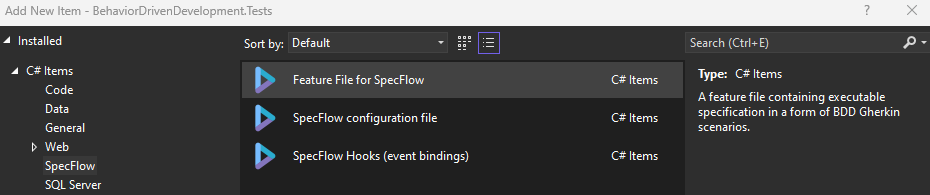And let’s define our feature using Gherkin syntax:

```Feature: Helpers
Provide numbers related helper methods

@Numbers
Scenario: Sum Two Numbers
Given the first number is 35
When the second number is 25
Then the result should be 60```

First, we define the feature name using the keyword Feature. Below is a short description of the feature.

@Numbers is the tag definition. We can use tags to group and execute scenarios.

Next, we have a scenario definition. The scenario is a test case in a Given-And-When-Then format.  After the keyword Scenario, we define the scenario name. Then we have a Given or And section where we define our system state before we run the test. When section defines the action we perform, and finally, Then section defines the state of a system after the action.

We need to define SpecFlow step definitions for our scenario. Using the SpecFlow VS extension makes this easy. Right-click on the scenario definition and select the “Define Steps…” option.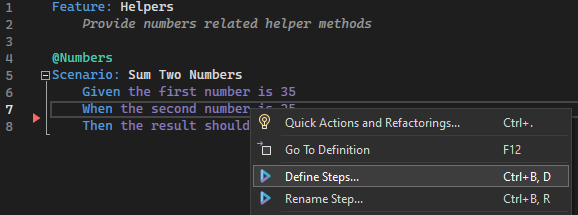The define steps window is opened, where we can copy the proposed steps definitions or create steps definition code immediately.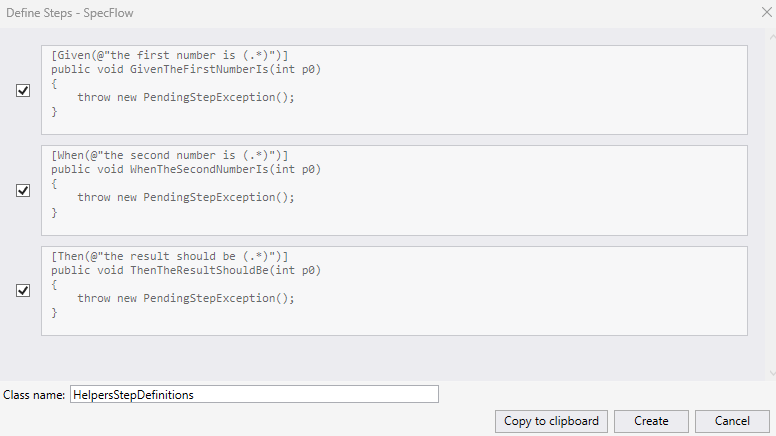This creates a placeholder code for us:

```[Binding]
{
[Given(@"the first number is (.*)")]
public void GivenTheFirstNumberIs(int p0)
{
throw new PendingStepException();
}

[When(@"the second number is (.*)")]
public void WhenTheSecondNumberIs(int p0)
{
throw new PendingStepException();
}

[Then(@"the result should be (.*)")]
public void ThenTheResultShouldBe(int p0)
{
throw new PendingStepException();
}
}```

### Defining Our Logic

We need the code for the defined steps. First, let’s define the class constructor:

```ScenarioContext scenarioContext;

{
scenarioContext = context;
}
```

Here we defined `ScenarioContext` instance, which allows us to transfer data between scenario steps.

`ScenarioContext` is similar to the dictionary regarding how the data is accessed and modified.

Now we define our Given step:

```[Given(@"the first number is (\d+)")]
public void GivenTheFirstNumberIs(int p0)
{
scenarioContext["FirstNumber"] = p0;
}```

We changed the regular expression to allow only numbers and assigned the value of the input parameter to the FirstNumber property of the `ScenarioContext` object.

We do the same for the When step:

```[When(@"the second number is (\d+)")]
public void WhenTheSecondNumberIs(int p0)
{
scenarioContext["SecondNumber"] = p0;
}```

And finally, we define the logic for Then step:

```[Then(@"the result should be (\d+)")]
public void ThenTheResultShouldBe(int p0)
{
var result = Helpers.SumTwoNumbers((int)scenarioContext["FirstNumber"],
(int)scenarioContext["SecondNumber"]);

Assert.IsTrue(result == p0);
}```

We are using the Scenario Context data we added in previous steps as inputs for our `SumTwoNumber()` method. The expected result is parsed using the regular expression. Finally, we use `Assert` to validate our function result against the expected result.

Highlighting in the Helpers features file indicates all the scenario sections are covered with the step definitions. Our test method is created automatically.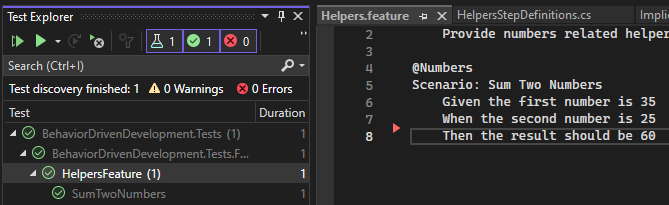### More Examples

Let’s see a couple of variations to the previous example.

First, we add a scenario describing the usage of `SubtractTwoNumber()` method:

```Scenario: Subtract Two Numbers
Given the first number is 35
When the second number is 25
Then the subtract result should be 10```

Since the Given and When sections are the same as in the first example, we only need to define the Then section step for this scenario:

```[Then(@"the subtract result should be (\d+)")]
public void ThenTheSubtractResultShouldBe(int p0)
{
var result = Helpers.SubtractTwoNumbers((int)scenarioContext["FirstNumber"],
(int)scenarioContext["SecondNumber"]);

Assert.IsTrue(result == p0);
}```

In this case, we are using `SubtractTwoNumbers()` method to get the result. The result is validated the same way.

In our following scenario, we are going to include And section:

```Scenario: Average of three numbers
Given the first number is 35
And the second number is 25
And the third number is 33
Then the average is 31```

We can notice there is no When section, and we have two lines with And keyword.

The first And line is the same as the When line in previous examples. That means we can add this case to the existing step definition:

```[When(@"the second number is (\d+)")]
[Given(@"the second number is (\d+)")]
public void WhenTheSecondNumberIs(int p0)
{
scenarioContext["SecondNumber"] = p0;
}```

And we only need to define the steps for the second And line and the result:

```[Given(@"the third number is (\d+)")]
public void GivenTheThirdNumberIs(int p0)
{
scenarioContext["ThirdNumber"] = p0;
}

[Then(@"the average is (\d+)")]
public void ThenTheAverageIs(int p0)
{
var result = Helpers.GetAverage((int)scenarioContext["FirstNumber"],
(int)scenarioContext["SecondNumber"],
(int)scenarioContext["ThirdNumber"]);

Assert.IsTrue(result == p0);
}```

In this example, we notice And step definition is the same as the Given step definition.

## Scenario Outline

Scenario Outline is a feature that allows us to use the same scenario for multiple combinations of inputs and outputs.

We are going to use the `Extensions`,  class we already defined to show how this can be useful.

We can define a simple scenario for the usage of `GetWordCount()` method:

```@StringExtensions
Scenario: Get Word Count
When the phrase is Behavior Driven Development
Then the word count is 3```

Step definitions for this scenario are:

```[When(@"the phrase is (.*)")]
public void WhenThePhraseIs(string phrase)
{
scenarioContext["Phrase"] = phrase;
}

[Then(@"the word count is (\d+)")]
public void ThenTheWordCountIs(int count)
{
var _count = ((string)scenarioContext["Phrase"]).GetWordCount();
Assert.IsTrue(count == _count);
}
```

This covers only one test case. But what if we want to test our method on multiple phrases?

This is where Scenario Outline comes to play. We use replace tags and Examples table to define different combinations:

```@StringExtensions
Scenario: Get Word Count
When the phrase is <phrase>
Then the word count is <count>

Examples:
| phrase                         | count |
| Behavior Driven Development    | 3     |
| Code-Maze articles are amazing | 4     |
| And I will subscribe for more  | 6     |```

<phrase> and <count> values are replaced with the corresponding sentences under the phrase column in the Examples table, and <count> value is replaced with the corresponding values under the count in the Examples table.

Each row in the examples table has its own test execution and result.

It is important to note we do not need to make any changes in the steps definitions. This saves a lot of time for the developers and enables testers to define additional test cases without involving the developer.

```Scenario: Get Charachter Count
When the phrase is <phrase>
Then the char count is <count>

Examples:
| phrase                         | count |
| Behavior Driven Development    | 25    |
| Code-Maze articles are amazing | 27    |
| And I will subscribe for more  | 24    |```

Here we have a different Then statement, so we need to define a step definition for it:

```[Then(@"the char count is (\d+)")]
public void ThenTheCharCountIs(int count)
{
var _count = ((string)scenarioContext["Phrase"]).GetCharCount();

Assert.IsTrue(count == _count);
}```

## Background Feature

We can use the Background feature to extract common sections in our scenarios, keeping our features definition clean and easy to maintain.

Let’s say we want to add the same prefix to every phrase we test against. Instead of adding it to all scenarios, we can use the Background feature:

```Feature: Extensions
Provide string extension methods

Background:
Given the phrase prefix is Phrase: .

Scenario: Get Word Count
When the phrase is <phrase>
Then the word count is <count>

Examples:
| phrase                         | count |
| Behavior Driven Development    | 4     |
| Code-Maze articles are amazing | 5     |
| And I will subscribe for more  | 7     |

Scenario: Get Charachter Count
When the phrase is <phrase>
Then the char count is <count>

Examples:
| phrase                         | count |
| Behavior Driven Development    | 32    |
| Code-Maze articles are amazing | 34    |
| And I will subscribe for more  | 31    |```

Here we also adjusted expected counts to consider the Prefix value.

In the steps definition, we need to define a step for the Prefix statement:

```[Given(@"the phrase prefix is (.*).")]
public void GivenThePhrasePrefixIsPhrase(string prefix)
{
scenarioContext["Prefix"] = prefix;
}```

And adjust our test methods to account for the Prefix value:

```[Then(@"the word count is (\d+)")]
public void ThenTheWordCountIs(int count)
{
var _count = (\$"{(string)scenarioContext["Prefix"]}{(string)scenarioContext["Phrase"]}")
.GetWordCount();

Assert.IsTrue(count == _count);
}

[Then(@"the char count is (\d+)")]
public void ThenTheCharCountIs(int count)
{
var _count = (\$"{(string)scenarioContext["Prefix"]}{(string)scenarioContext["Phrase"]}")
.GetCharCount();

Assert.IsTrue(count == _count);
}
```

## Conclusion

In this article, we learned the bases of Behavior Driven Development methodology. We also learned how to install and use SpecFlow and saw different features in action through our examples.

We conclude this article by noting that BDD is not a tool, a new approach to development, or a new syntax. It combines all these components and offers great benefits to the team if good team collaboration is in place. If you want to learn more about BDD, we suggest an excellent article on the SpecFlow blog by J.B.Rainsberger.